## Block Diagram Reduction

In the figure below, simplify/reduce the block diagram to a single element that relates the input to the output. Write your answer in transfer function form.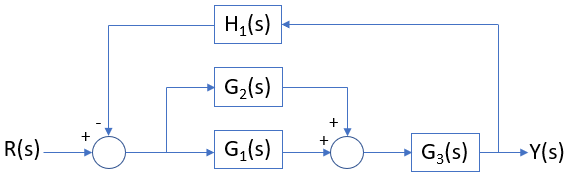Hint
If the blocks are connected in series: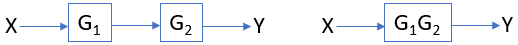Hint 2
If the blocks are connected in parallel: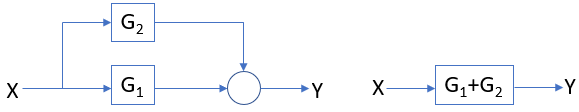Rules for block diagram reduction:
• If the blocks are connected in series:• If the blocks are connected in parallel:• If the blocks are connected in feedback loop: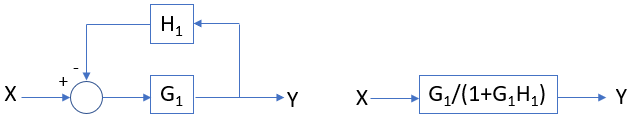Thus, we can break down the block diagram into three sections (parallel, series, and feedback loop). Starting with parallel: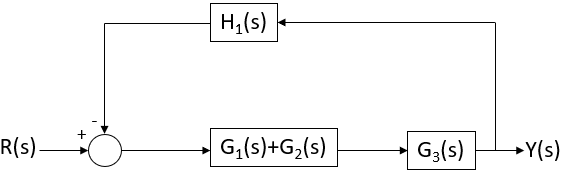For the series: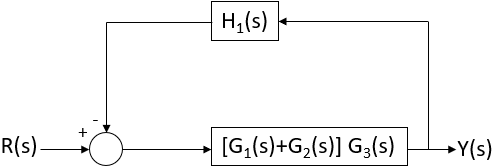Finally for the feedback loop: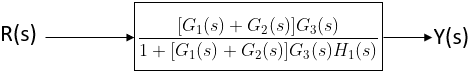Formatting the answer in transfer function form:
$$\frac{Y(s)}{R(s)}=\frac{[G_1(s)+G_2(s)]G_3(s)}{1+[G_1(s)+G_2(s)]G_3(s)H_1(s)}$$$$$\frac{Y(s)}{R(s)}=\frac{[G_1(s)+G_2(s)]G_3(s)}{1+[G_1(s)+G_2(s)]G_3(s)H_1(s)}$$$